Now that the modules have been placed in the matrix, the best mask pattern must be determined. A mask pattern changes which modules are dark and which are light according to a particular rule. The purpose of this step is to modify the QR code to make it as easy for a QR code reader to scan as possible.

If a module in the QR code is "masked", this simply means that if it is a light module, it should be changed to a dark module, and if it is a dark module, it should be changed to a light module. In other words, masking simply means to toggle the color of the module.

The QR code specification defines eight mask patterns that can be applied to the QR code. For example, for mask pattern #1, every even-numbered row in the QR matrix is masked, and for mask pattern #2, every third column in the QR matrix is masked.

Mask patterns must ONLY be applied to data modules and error correction modules. In other words:

• Do not mask function patterns (finder patterns, timing patterns, separators, alignment patterns)
• Do not mask reserved areas (format information area, version information area)

After a mask pattern has been applied to the QR matrix, it is given a penalty score based on four evaluation conditions that are defined in the QR code specification. A QR code encoder must apply all eight mask patterns and evaluate each one. Whichever mask pattern results in the lowest penalty score is the mask pattern that must be used for the final output.

#### How to Evaluate Reserved Areas

Note that the entire matrix (including function patterns and reserved areas) is evaluated, even though the masking is only applied to data and error correction modules.

#### The Four Penalty Rules

The four penalty rules can be summarized as follows:

• The first rule gives the QR code a penalty for each group of five or more same-colored modules in a row (or column).
• The second rule gives the QR code a penalty for each 2x2 area of same-colored modules in the matrix.
• The third rule gives the QR code a large penalty if there are patterns that look similar to the finder patterns.
• The fourth rule gives the QR code a penalty if more than half of the modules are dark or light, with a larger penalty for a larger difference.

#### Evaluation Condition #1

For the first evaluation condition, check each row one-by-one. If there are five consecutive modules of the same color, add 3 to the penalty. If there are more modules of the same color after the first five, add 1 for each additional module of the same color. Afterward, check each column one-by-one, checking for the same condition. Add the horizontal and vertical total to obtain penalty score #1.

The following images illustrate the process of evaluating the QR code in this manner. In this example, the horizontal penalty is 92, and the vertical penalty is 88. Therefore, penalty score #1 is 92 + 88 = 180.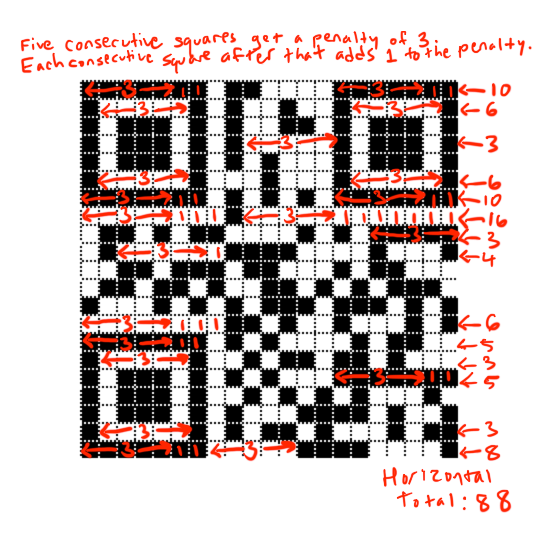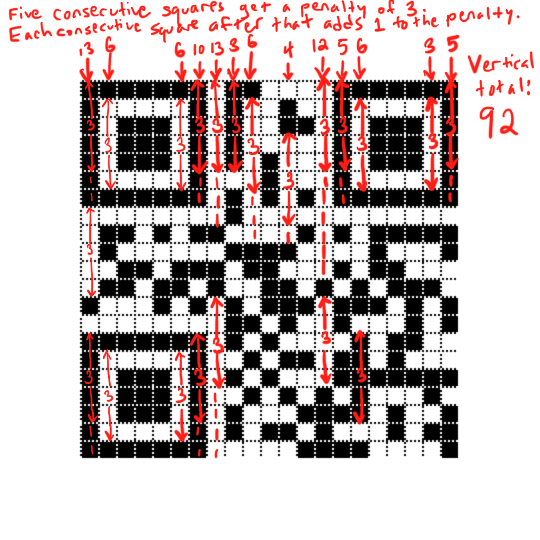#### Evaluation Condition #2

For second evaluation condition, look for areas of the same color that are at least 2x2 modules or larger. The QR code specification says that for a solid-color block of size m × n, the penalty score is 3 × (m - 1) × (n - 1). However, the QR code specification does not specify how to calculate the penalty when there are multiple ways of dividing up the solid-color blocks.

Therefore, rather than looking for solid-color blocks larger than 2x2, simply add 3 to the penalty score for every 2x2 block of the same color in the QR code, making sure to count overlapping 2x2 blocks. For example, a 3x2 block of the same color should be counted as two 2x2 blocks, one overlapping the other.

The following image illustrates how to calculate penalty rule #2.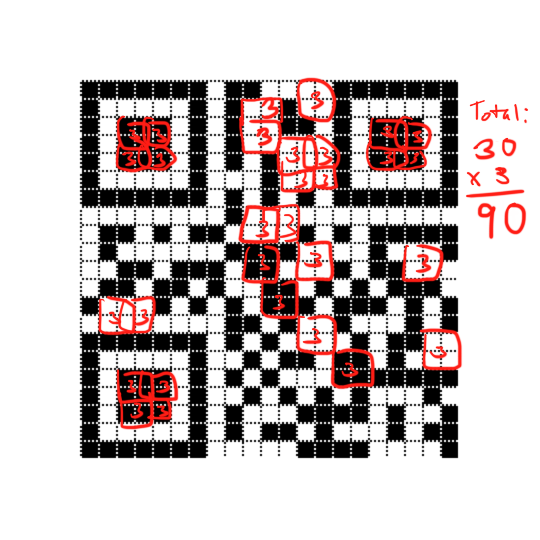#### Evaluation Condition #3

The third penalty rule looks for patterns of dark-light-dark-dark-dark-light-dark that have four light modules on either side. In other words, it looks for any of the following two patterns: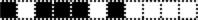OR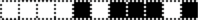Each time this pattern is found, add 40 to the penalty score. In the example below, there are two such patterns. Therefore, penalty score #3 is 80.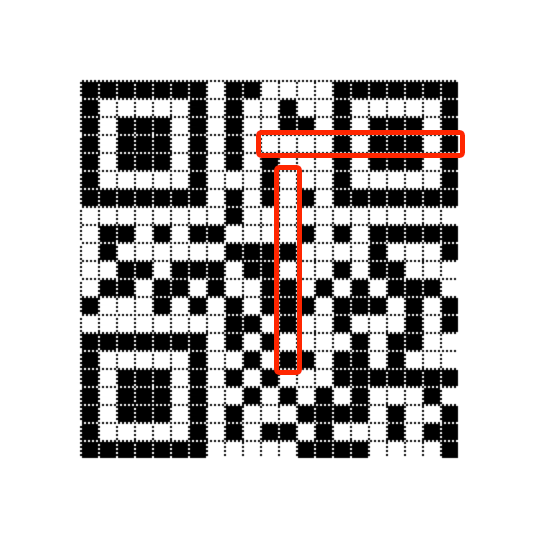#### Evaluation Condition #4

The final evaluation condition is based on the ratio of light modules to dark modules. To calculate this penalty rule, do the following steps:

1. Count the total number of modules in the matrix.
2. Count how many dark modules there are in the matrix.
3. Calculate the percent of modules in the matrix that are dark: (darkmodules / totalmodules) * 100
4. Determine the previous and next multiple of five of this percent. For example, for 43 percent, the previous multiple of five is 40, and the next multiple of five is 45.
5. Subtract 50 from each of these multiples of five and take the absolute value of the result. For example, |40 - 50| = |-10| = 10 and |45 - 50| = |-5| = 5.
6. Divide each of these by five. For example, 10/5 = 2 and 5/5 = 1.
7. Finally, take the smallest of the two numbers and multiply it by 10. In this example, the lower number is 1, so the result is 10. This is penalty score #4.

For another example, in the image below, the total number of modules is 441, and the total number of dark modules is 213.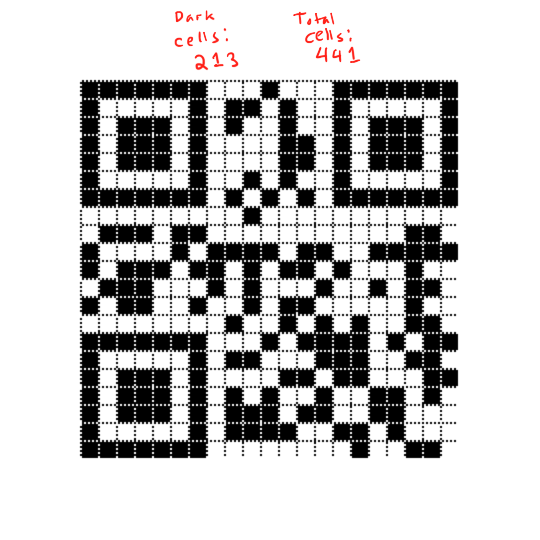The percent of dark modules is (213 / 441) * 100 ≈ 48.2993

The previous multiple of five is 45 and the next multiple of five is 50.

Subtract by 50 and take the absolute value of each:
|45 - 50| = |-5| = 5
|50 - 50| = 0

Divide each by five:
5/5 = 1
0/5 = 0

The smallest of those two numbers is 0. Multiplied by 10, this is still 0. Therefore, penalty score #4 is 0 in this example.

#### Add the Four Penalty Scores

To complete the evaluation of a QR code, add the four penalty scores. The total is the overall penalty score of the QR code.

### Choose the Lowest Penalty Score for the Eight Mask Patterns

The following images show eight QR codes, one for each mask pattern. All eight QR codes in this example encode the same data. As shown, the mask pattern with the lowest penalty score is mask pattern 0. Therefore, in this example, the QR encoder should use mask pattern 0 when outputting the final QR code.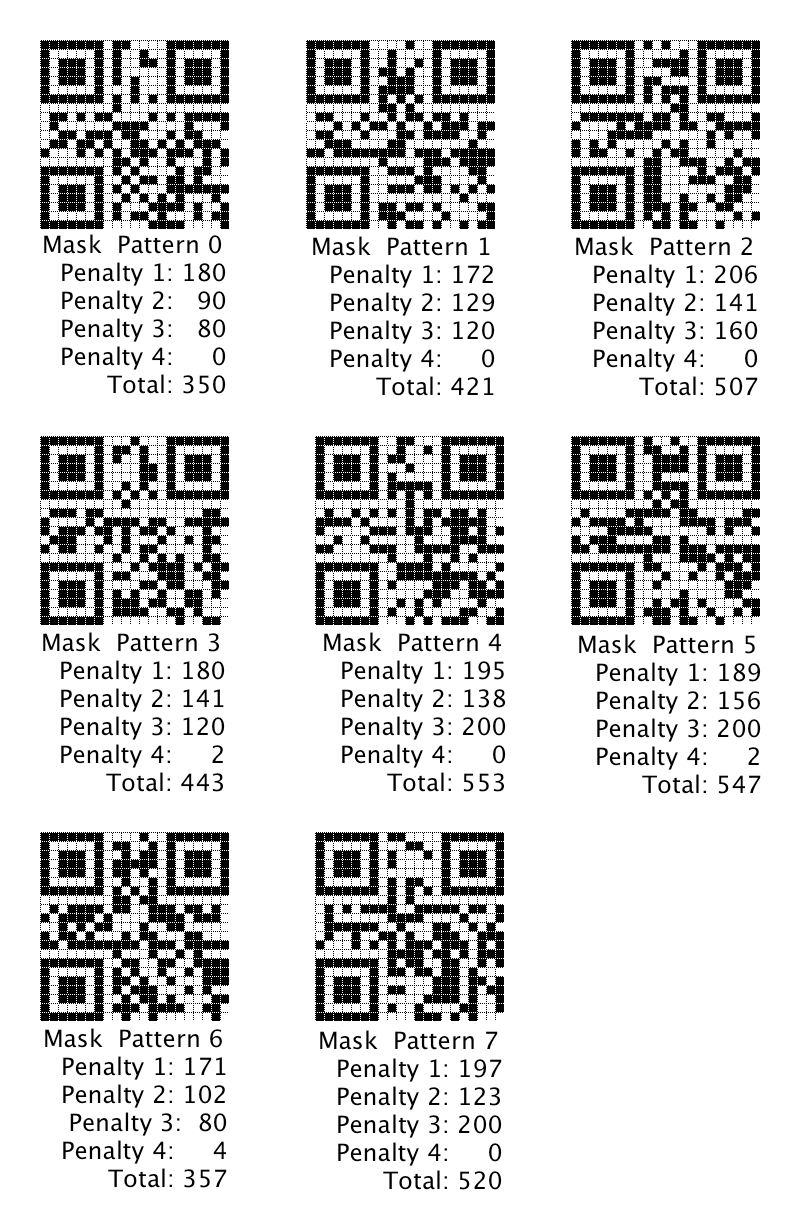### Next: Add Format and Version Information

When adding the data and error correction modules, the format and version areas were reserved for later use. The final step of encoding a QR code is to add the format and version information to the QR code.